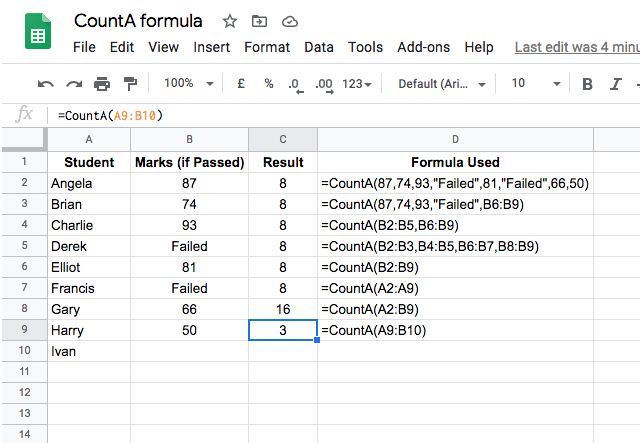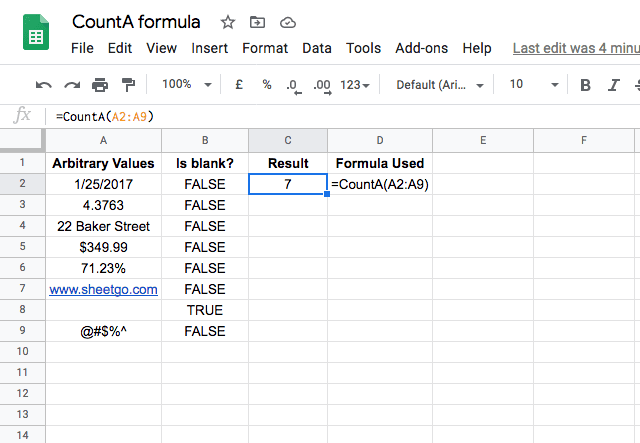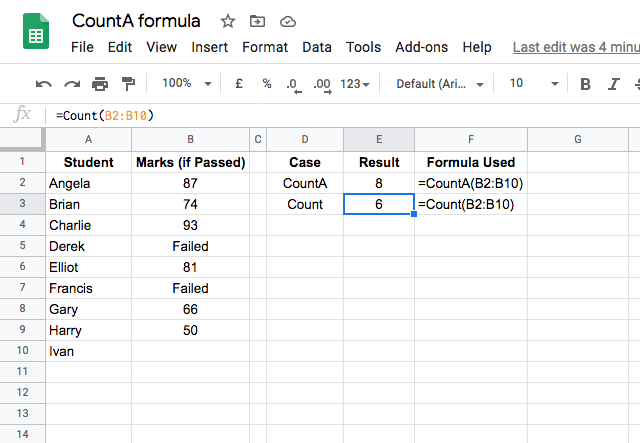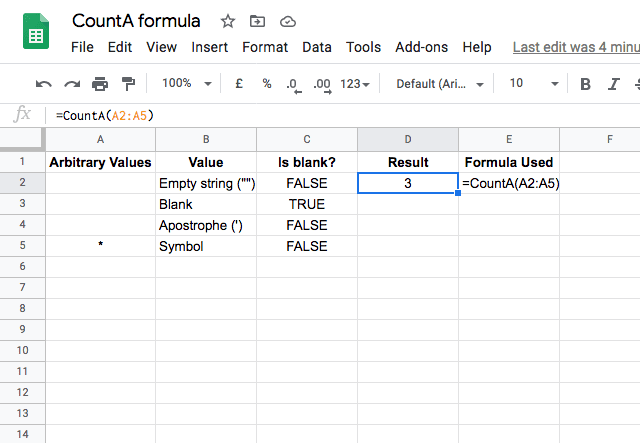# How to use the COUNTA function in Google SheetsThe COUNTA function in Google Sheets is a simple and widely-used function for everyday spreadsheet work. It quickly gives you the total number of values within a specific range of cells. Whereas the COUNT function only counts cells containing numbers, the COUNTA function counts how many cells in the dataset contain any kind of data. That includes numerical values, text (including white space and zero-length strings), logical expressions, error messages, dates and formulas.

Repeated values are also counted. Blank or empty cells are excluded.

## Syntax

=COUNTA(value1, [value2, …])
• value1 – the value or range of cell(s) to count within.
• value2, value3 … – (optional, up to 30) additional values to also be included in the count.

The value arguments can be:

• A value
• A cell reference
• A range of cells
• A named range
How to use COUNTA function

I will show the function in action with a few examples:You can see that the input parameters can take many forms: a number, text within double quotes, and a reference to a range of cells The most basic form of the function uses a range as an input with the formula =CountA(B2:B9).

No matter what kind of combination you use for the input parameters, the COUNTA function does its job of counting the values. In all of these cases from row 2 through to 7, the function returned the result 8, as there are 8 different values in the third column (including the “Failed” ones). Therefore, you can see that the function counts both numbers and text values.

I included two column ranges in the case in row 8, hence the result was 16. But in the final example, you will see that the output returned is 3, while the data set consists of values from 4 cells: A9, A10, B9 and B10. This difference is because the COUNTA function doesn’t count blank values

COUNTA function also counts other types of data:In the example above, I entered various arbitrary values like a date, currency, percentage, decimal, and general text and even special characters. I also validated for blank values on the second column, using the ISBLANK function. So, all the values in rows 2 through to 9 (barring row 8) are non-blank values. Not surprisingly, the COUNTA function in column C counted all the non-blank values, and gives a total count of 7.

### COUNTA vs. COUNT

The COUNT function in Google Sheets is similar to COUNTA, except COUNT only counts numbers, whereas COUNTA counts all values. The example below highlights the difference between the two.Here I use both functions on the same set of data from the first example. You can see that the COUNTA function counts both the numerical values, and the text values for the students that failed. The COUNT function, on the other hand, only counts the numerical values and ignores the text values. Neither function counts the blank cell for Ivan’s test score.

### Blank cells

By now you understand that the COUNTA function counts all cells that contain a value, and ignores any cell that is blank. However, there are some tricky cases where a cell can appear blank but actually has a value and is included in the count. The example below illustrates this.At first glance, it looks like the first three cells in the list are all blank. However, from the Is Blank? column, you can see that only the second cell is truly blank. The others are an empty string, and an apostrophe, both of which appear to be blank but are not. When you use the COUNTA function, these cells are counted – so keep this in mind when using the function!

Want to count how many unique values appear in a range? Use Google Sheets COUNTUNIQUE function instead.

Editor’s note: This is a revised version of a previous post that has been updated for accuracy and comprehensiveness.